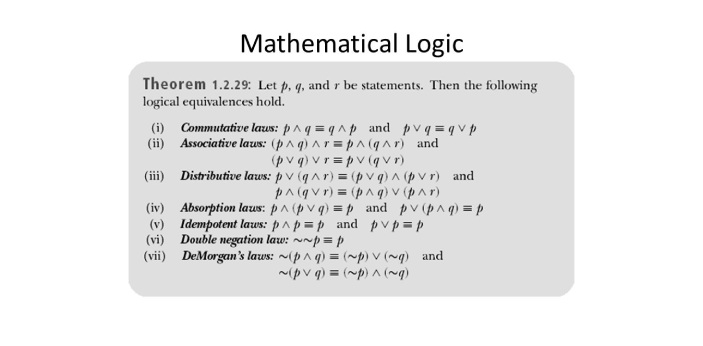How much would he have spent on gasoline in 2 weeks getting back and - ProProfs DiscussTopicsMoreProductsMore+ Ask Question

# How much would he have spent on gasoline in 2 weeks getting back and forth to work? John works 4 days per week. He drives 10 miles round trip per day. Gas is \$2.50 per gallon and his car gets 20 miles to the gallon.

A. \$8.50
B. \$10.00
C. \$12.00
D. \$9.50

This question is part of Logic and Math Quiz
Asked by Geotilley, Last updated: Apr 05, 2020

###Request 0FollowShareAnswer AnonymouslyAnswer LaterCopy Link#### E. Stanley

E. Stanley, Technical writer, Indianapolis

The correct answer to this question is B, \$10. This question would mainly be found on a math test, focusing on mathematical logic. This logic uses many theories including set, model, recursion, and proof. The study of mathematical logic began in 19th century and was then used in areas of geometry and analysis.Beyond math, mathematical logic can be used in fields such as law, economics, and history. There are many types of mathematical logics, such as first order logic which uses formulas as an expression. There is also infinitary logic, which uses formulas to provide an infinite amount of information.#### John Smith

John Smith\$10.00Search for Google imagesSelect a recommended image
Upload from your computerCancelSearch for Google imagesSelect a recommended image
Upload from your computerCancelSearch for Google imagesSelect a recommended image
Upload from your computerCancel